# Choose the Symbol that Follows the Rule

In this worksheet, students will match pairs of shape-based symbols in the same way as a pair of examples. It will develop their ability to categorise and use skills such as rotation and mirror imaging.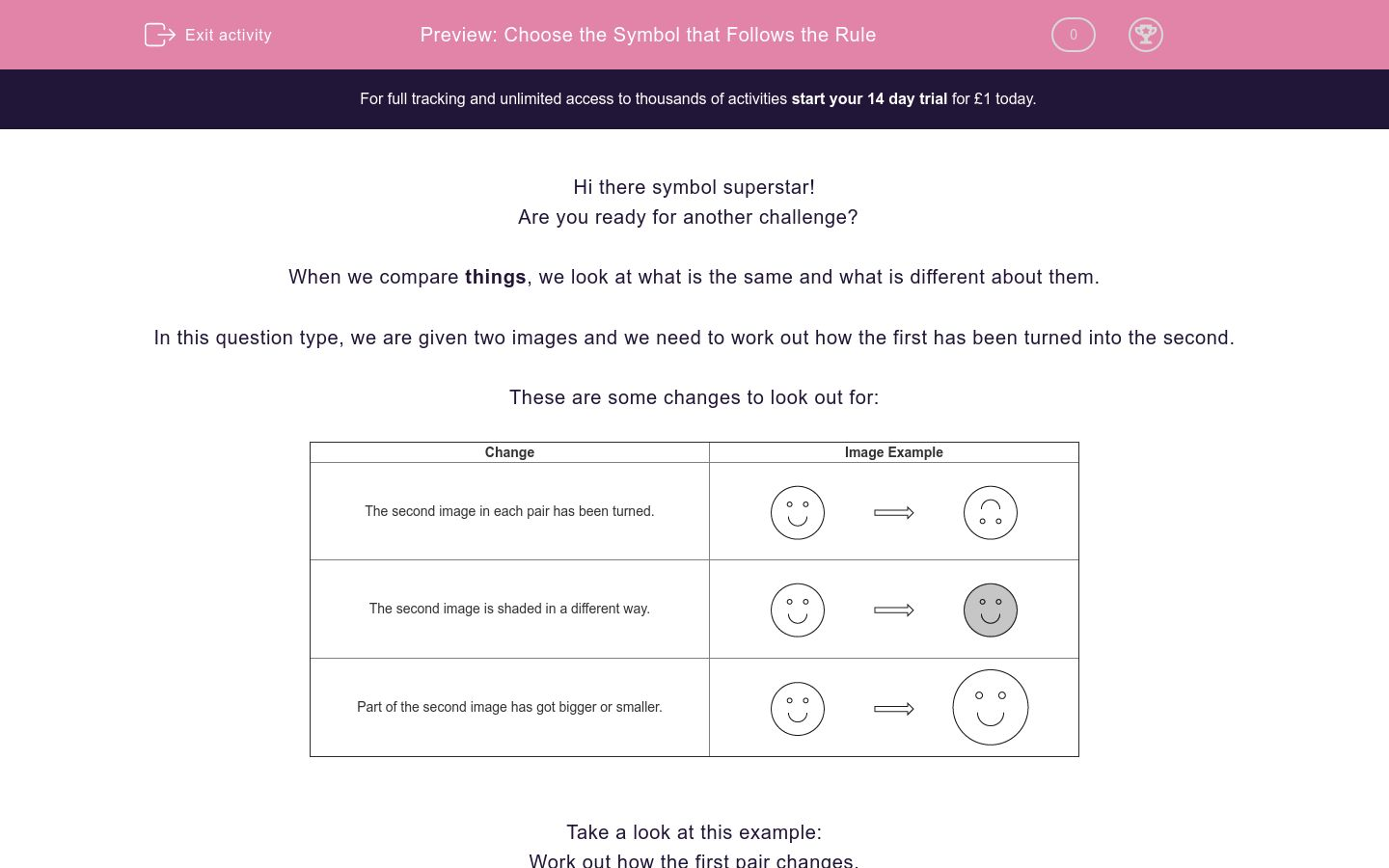Key stage:  KS 2

Curriculum topic:  Spatial and Non-Verbal Reasoning

Curriculum subtopic:  Apply the Relationship

Difficulty level:### QUESTION 1 of 10

Hi there symbol superstar!

Are you ready for another challenge?

When we compare things, we look at what is the same and what is different about them.

In this question type, we are given two images and we need to work out how the first has been turned into the second.

These are some changes to look out for:

 Change Image Example The second image in each pair has been turned.The second image is shaded in a different way.Part of the second image has got bigger or smaller.Take a look at this example:

Work out how the first pair changes.

Then use the same rule to work out which image best completes the second pair.Which of these options would the square turn into?

a)b)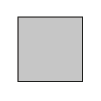c)Did you notice that the shading is darker between the first and second images in the first pair?

Therefore, we must also make the shading darker in the second pair, so the answer which best fits the sequence is b.

In this activity, you will need to be a symbol superstar and match images based on a pattern that you will find.

Remember to work out how the first image changes into the second image to find the rule.

Let’s get started!

Look at the first pair of images here.

Can you see how they are related?

Apply this relationship to the second set by choosing the image from the options below which would best complete the pair.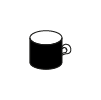Look at the first pair of images here.

Can you see how they are related?

Apply this relationship to the second set by choosing the image from the options below which would best complete the pair.Look at the first pair of images here.

Can you see how they are related?

Apply this relationship to the second set by choosing the image from the options below which would best complete the pair.Look at the first pair of images here.

Can you see how they are related?

Apply this relationship to the second set by choosing the image from the options below which would best complete the pair.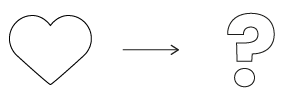Look at the first pair of images here.

Can you see how they are related?

Apply this relationship to the second set by choosing the image from the options below which would best complete the pair.Look at the first pair of images here.

Can you see how they are related?

Apply this relationship to the second set by choosing the image from the options below which would best complete the pair.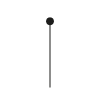Look at the first pair of images here.

Can you see how they are related?

Apply this relationship to the second set by choosing the image from the options below which would best complete the pair.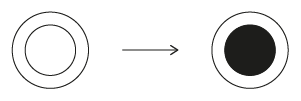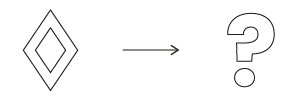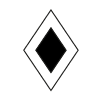Look at the first pair of images here.

Can you see how they are related?

Apply this relationship to the second set by choosing the image from the options below which would best complete the pair.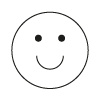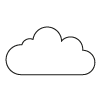Look at the first pair of images here.

Can you see how they are related?

Apply this relationship to the second set by choosing the image from the options below which would best complete the pair.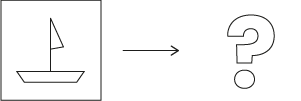Look at the first pair of images here.

Can you see how they are related?

Apply this relationship to the second set by choosing the image from the options below which would best complete the pair.• Question 1

Look at the first pair of images here.

Can you see how they are related?

Apply this relationship to the second set by choosing the image from the options below which would best complete the pair.EDDIE SAYS
This question focuses on the size of the images. The bones has been made smaller so we need to do the same thing to the mug. This means that option a is the best fit for the second pair. Try not to get caught out by option c, as it has been shaded as well even though it is the correct size. Both of these are probably the size of mug that an ant would drink out of!
• Question 2

Look at the first pair of images here.

Can you see how they are related?

Apply this relationship to the second set by choosing the image from the options below which would best complete the pair.EDDIE SAYS
Did you get this one right symbol detective? This is a rotation-themed question. The first image has been rotated a quarter of a turn (or 90 degrees) clockwise. We need to do the same with the heart, which makes option b the best fit.
• Question 3

Look at the first pair of images here.

Can you see how they are related?

Apply this relationship to the second set by choosing the image from the options below which would best complete the pair.EDDIE SAYS
I wish every day looked as sunny as this question! The clue is in the image here - we need to look at the shading or brightness to reach the correct answer. The first pair of images have moved from white to lightly shaded. We need to do the same with the sunshine image. This makes option a the best fit in the second pair. Don't get caught out by option b, as it is too dark or heavily shaded.
• Question 4

Look at the first pair of images here.

Can you see how they are related?

Apply this relationship to the second set by choosing the image from the options below which would best complete the pair.EDDIE SAYS
Did you spot the clue in this one detective? This is similar to the other shading question but involves a pattern rather than a solid colour. The second drinking cup in the pair has had a stripy pattern added to it. We need to do the same with the heart and add a stripy pattern. This makes option b the best fit in the second pair.
• Question 5

Look at the first pair of images here.

Can you see how they are related?

Apply this relationship to the second set by choosing the image from the options below which would best complete the pair.EDDIE SAYS
Here's another question where you need to focus on size. In the first pair, the apple has become much bigger. We need to do the same with the square in the second pair. This makes option c the correct answer. You're doing great! Keep going detective!
• Question 6

Look at the first pair of images here.

Can you see how they are related?

Apply this relationship to the second set by choosing the image from the options below which would best complete the pair.EDDIE SAYS
This is a rotation-themed question. The arrow in the first pair has been rotated by a half turn or 180 degrees. We need to do the same to the lollipop image. This makes option a the best fit in the second pair. Well done if you spotted the clue here detective! Now, let's try another one.
• Question 7

Look at the first pair of images here.

Can you see how they are related?

Apply this relationship to the second set by choosing the image from the options below which would best complete the pair.EDDIE SAYS
For this question, you need to look at the shading but there are two layers to consider. In the first pair, the middle section has been shaded but the outer edge has remained unchanged. If we apply this change to the second pair, we find that option b is the best fit as it has the middle section shaded but kept the same outer edge. Keep up the hard work super shader!
• Question 8

Look at the first pair of images here.

Can you see how they are related?

Apply this relationship to the second set by choosing the image from the options below which would best complete the pair.EDDIE SAYS
Here the smiley face has become much larger. This makes me extra happy! We need to apply the same change to the cloud, making option c the best fit in the second pair.
• Question 9

Look at the first pair of images here.

Can you see how they are related?

Apply this relationship to the second set by choosing the image from the options below which would best complete the pair.EDDIE SAYS
Here the triangle has been rotated a quarter turn (or 90 degrees) to the right or in a clockwise direction. So we need to do the same with the boat in the second pair. This makes option c the best fit. I hope this boat doesn't sink! It would certainly be very tricky to sail at this orientation!
• Question 10

Look at the first pair of images here.

Can you see how they are related?

Apply this relationship to the second set by choosing the image from the options below which would best complete the pair.